Next: Constraining the mass of Up: Unbiased results Previous: Unbiased results   Contents

## Uniform prior and fictitious quantities

Let us consider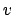independent data sets, or experiments, each of which gives information on the quantity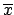. For each data set there is a likelihood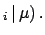data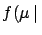Each data set gives, by itself, the following information: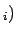data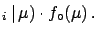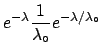data(9.14)

The global inference is obtained using Bayes' theorem iteratively: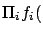data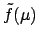data(9.15)

We may use, as a formal tool, a ficticious inference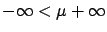using for each data set a uniform prior in the range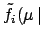: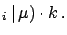datadata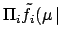(9.16)

This allows us to rewritedatadata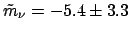This stratagem has the advantage that one can report pseudoresults' on fictitious quantities which, in the case of Gaussian likelihoods, may be combined according to the usual formula of the average with the inverse of the variances (see Section). They can be transformed, finally, into the physical result using the physical prior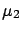. It is important to state the procedure clearly and, if possible, to indicate the fictitious quantity with different symbols. For example, the result of the problem of Sectioncan be reported in the following way:
From the observed value of -5.4eV and the knowledge of the likelihood, described by a normal distribution centred in the true value of the mass with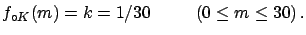eV independent of the mass, we get a fictitious mass of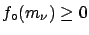eV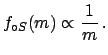where fictitious' indicates a hypothetical mass which could assume any real number with uniform distribution. Assuming the more physical hypothesis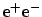yields to ...(see figure ...), from which follows a 95% upper limit of 3.9eV.'' The conclusion of this section is that the uniform prior is a convenient prior for many purposes:
• it produces results very similar to those obtainable using the rational priors of those who have done the experiment, as shown in many of the examples given in these notes (see, for example, Section);
• it allows easy combination of data and a physics motivated prior can be added at the end;
• there is no problem of `double counting' the same prior, as would happen if several experimenters were to use the same non-uniform prior to infer the same quantity from different data.
The problem of presenting unbiased results in frontier measurements is also discussed in Refs. , ,  and .Next: Constraining the mass of Up: Unbiased results Previous: Unbiased results   Contents
Giulio D'Agostini 2003-05-15